Courses

# Integer Value of Moving Charges and Magnetism the Following, of Moving Charges and Magnetism , JEE Notes | EduRev

## Class 12 Physics 35 Years JEE Mains &Advance Past year Paper

Created by: Abhishek Kapoor

## JEE : Integer Value of Moving Charges and Magnetism the Following, of Moving Charges and Magnetism , JEE Notes | EduRev

The document Integer Value of Moving Charges and Magnetism the Following, of Moving Charges and Magnetism , JEE Notes | EduRev is a part of the JEE Course Class 12 Physics 35 Years JEE Mains &Advance Past year Paper.
All you need of JEE at this link: JEE

Q.1. A steady current I goes through a wire loop PQR having shape of a right angle triangle with PQ = 3x, PR = 4x and QR = 5x. If the magnitude of the magnetic field at P due to this loop is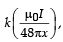find the value of k.

Ans. 7

Solution.

The right angled triangle is shown in the figure. Let us drop a perpendicular from P on QR which cuts QR at M.

The magnatic field due to currents in PQ and RP at P is zero.

The magnetic field due to current in QR at P is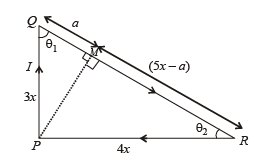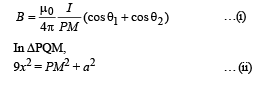In ΔPRM,

16x2 = PM2 + (5x – a)2 … (iii)

⇒ 7 x2 = 25 x2- 10xa ⇒  10xa =  18x2

a = 1.8 x … (iv)

From (ii) & (iv),

9 x2 = PM2+ (1.8x)2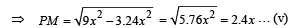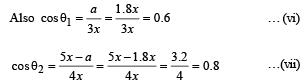From (i), (v), (vi) and (vii),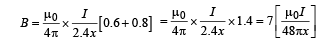Comparing it with B =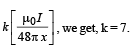Q.2. A long circular tube of length 10 m and radius 0.3 m carries a current I along its curved surface as shown. A wire-loop of resistance 0.005 ohm and of radius 0.1 m is placed inside the tube with its axis coinciding with the axis  of the tube. The current varies as I = I0cos(300 t) where I0 is constant. If the magnetic moment of the loop is Nμ0I0sin (300 t), then ‘N’ is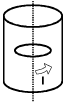Ans. 6

Solution. Let us consider an amperian loop ABCD which is a rectangle as shown in the figure. Applying ampere’s circuital law we get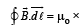(current passing through the loop)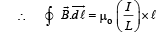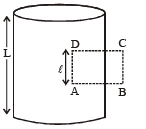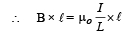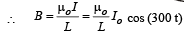The magnetic moment of the loop = (current in the loop) × πr2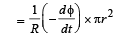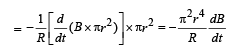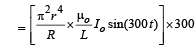Comparing it with the expression given in the question we get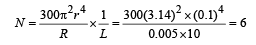Q.3.A cylindrical cavity of diameter a exists inside a cylinder of diameter 2a as shown in the figure. Both the cylinder and the cavity are infinity long. A uniform current density J flows along the length. If the magnitude of the magnetic field at the point P is given by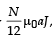then the value of N is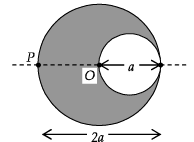Ans.5

Solution. Current density J =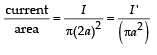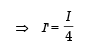Let us consider the cavity to have current I' flowing in both the directions.

The magnetic field at P due to the current flowing through the cylinder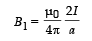The magnetic field at P due to the current (I’) flowing in opposite direction is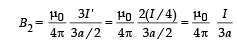∴ The net magnetic field is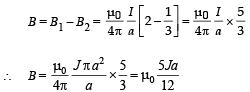Q.4. Two parallel wires in the plane of the paper are distance X0 apart. A point charge is moving with speed u between the wires in the same plane at a distance X1 from one of the wires. When the wires carry current of magnitude I in the same direction, the radius of curvature of the path of the point charge is R1. In contrast, if the currents I in the two wires have directions opposite to each other, the radius of curvature of the path is R2. If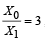the value of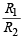is

Ans. 3

Solution.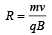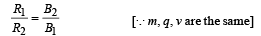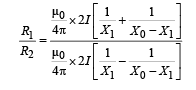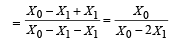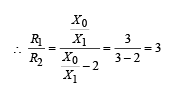Offer running on EduRev: Apply code STAYHOME200 to get INR 200 off on our premium plan EduRev Infinity!

42 docs|19 tests

,

,

,

,

,

,

,

,

,

,

,

,

,

,

,

,

,

,

,

,

,

,

,

,

,

,

,

;